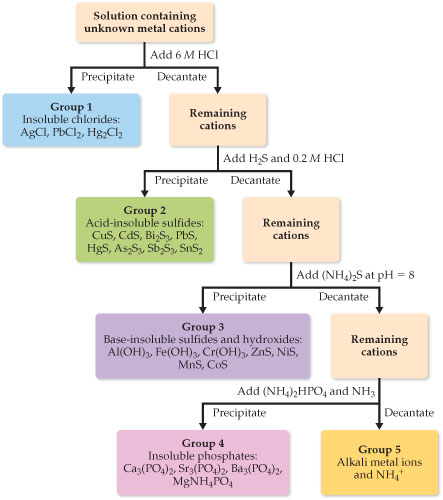# Problem: Qualitative analysis. A flowchart showing a common scheme for identifying cations.If a solution contained a mixture of Ag+ and Al3+ ions, would this separation scheme work?

###### FREE Expert Solution

We are asked if this separation scheme would work if solution contained mixture of Ag+ and Al3+ ions.

Cations are positively charged ions. The cations in the solution can be separated by precipitating one out.

We will refer to the solubility rules in order to determine if the scheme work to separate Ag+ and Al3+ ions in a mixture.

Solubility Rules:

Soluble Ionic Compounds:

Group 1A ions (Li+, Na+, K+, etc.) and Ammonium ion (NH4+) are soluble
Nitrates (NO3-), Acetates (CH3COO- or C2H3O2-), and most Perchlorates (ClO4-) are soluble
Cl-, Br-, and I- are soluble except when paired with Ag+, Pb2+, Cu+, Hg22+
Sulfates (SO42-) are soluble except those of Ca2+, Sr2+, Ba2+, Ag+, and Pb2+

• Insoluble Ionic Compounds:

88% (320 ratings)###### Problem Details

Qualitative analysis. A flowchart showing a common scheme for identifying cations.If a solution contained a mixture of Ag+ and Al3+ ions, would this separation scheme work?